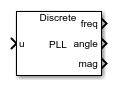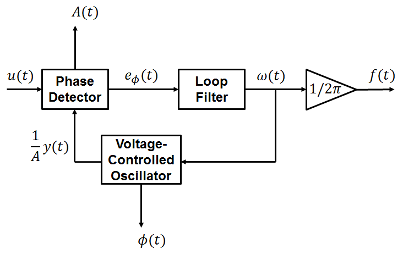# Sinusoidal Measurement (PLL)

Estimate sinusoidal characteristics using a phase-locked loop

•Libraries:
Simscape / Electrical / Control / Measurements

## Description

The Sinusoidal Measurement (PLL) block estimates the frequency, phase angle, and magnitude of a single-phase sinusoidal signal or individual phases of a multiphase sinusoidal signal. The block uses an enhanced phase-locked loop (PLL) strategy to estimate these sinusoidal characteristics of the input signal.

Use this block in control applications when the frequency, phase angle, or magnitude is required and cannot be measured directly. To provide faster phase locking for balanced three-phase input signals, use the Three-Phase Sinusoidal Measurement (PLL) block.

### Equations

The phase-locked loop generates a sinusoid that approximates the input signal u(t) with the form:

`$y\left(t\right)=A\left(t\right)\mathrm{sin}\left({\varphi }_{0}+\int 2\pi f\left(t\right)dt\right),$`

where:

• y is the estimate of the input signal.

• A is the estimate of the amplitude of the input signal.

• ϕ0 is the initial phase angle of the input signal.

The estimated phase angle ϕ is the angle of this generated sinusoid:

`$\varphi \left(t\right)={\varphi }_{0}+\int 2\pi f\left(t\right)dt,$`

where f if the frequency of the sinusoid, and ϕ0 is the initial phase angle.

This diagram shows the overall structure of the phase-locked loop.In the diagram:

• The phase detector produces an error signal relative to the phase difference eϕ between the input sinusoid u and the synthesized sinusoid y. It also outputs an estimate of the amplitude A.

• The loop filter provides an estimate of the input angular frequency ω by filtering out the high-frequency components of the phase difference. The block also outputs the converted frequency f in Hz.

• The voltage-controlled oscillator integrates the angular speed to produce the phase estimate ϕ. The oscillator also generates the normalized synthesized sinusoid (1/A)y which it sends to the Phase Detector for comparison.

## Ports

### Input

expand all

Periodic input signal.

Data Types: `single` | `double`

### Output

expand all

Estimated frequency of the input signal, in Hz.

Data Types: `single` | `double`

Estimated phase angle of the input signal, in rad.

Data Types: `single` | `double`

Estimated magnitude of the input signal.

Data Types: `single` | `double`

## Parameters

expand all

Integral gain for the phase detector. This determines the aggressiveness of the PLL in tracking and locking to the magnitude.

If the input signal is a vector, use scalar parameters or use vector parameters that are the same size as the input signal.

Proportional gain for the loop filter. This determines the aggressiveness of the PLL in tracking and locking to the phase angle. Increase this value to improve reaction time of the tracking to step changes in the phase angle.

If the input signal is a vector, use scalar parameters or use vector parameters that are the same size as the input signal.

Integral gain for the loop filter. Increase this value to increase the rate at which steady-state error is eliminated in the phase angle. This value also determines the aggressiveness of the PLL in tracking and locking to the phase.

If the input signal is a vector, use scalar parameters or use vector parameters that are the same size as the input signal.

Initial estimate of the input frequency. If the input signal is a vector, use scalar parameters or use vector parameters that are the same size as the input signal.

Initial estimate of the phase angle. If the input signal is a vector, use scalar parameters or use vector parameters that are the same size as the input signal.

Initial estimate of the magnitude. If the input signal is a vector, use scalar parameters or use vector parameters that are the same size as the input signal.

Time between consecutive block executions. During execution, the block produces outputs and, if appropriate, updates its internal state. For more information, see What Is Sample Time? and Specify Sample Time.

For inherited discrete-time operation, set this parameter to `-1`. For discrete-time operation, set this parameter to a positive integer. For continuous-time operation, set this parameter to `0`.

If this block is in a masked subsystem or a variant subsystem that supports switching between continuous operation and discrete operation, promote this parameter to ensure correct switching between the continuous and discrete implementations of the block. For more information, see Promote Block Parameters on a Mask.

 Karimi-Ghartemani, M., and M. R. Iravani. "A New Phase-Locked Loop (PLL) System." IEEE Transactions on Industrial Electronics. Proceedings of the 44th IEEE Symposium on Circuits and Systems, vol. 1, pp. 421-424. IEEE, 2001..